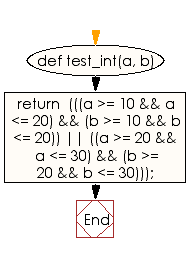﻿ Ruby Basic exercises: Check two integer values and return true if they are both in the range 10..20 - w3resource# Ruby Basic Exercises: Check two integer values and return true if they are both in the range 10..20

## Ruby Basic: Exercise-37 with Solution

Write a Ruby program to check two integer values and return true if they are both in the range 10..20 inclusive, or they are both in the range 20..30 inclusive.

Ruby Code:

``````def test_int(a, b)
return  (((a >= 10 && a <= 20) && (b >= 10 && b <= 20)) ||
((a >= 20 && a <= 30) && (b >= 20 && b <= 30)));
end

print test_int(10, 15),"\n"
print test_int(6, 9),"\n"
print test_int(22, 30),"\n"
print test_int(45, 55)
``````

Output:

```true
false
true
false
```

Flowchart:Ruby Code Editor: